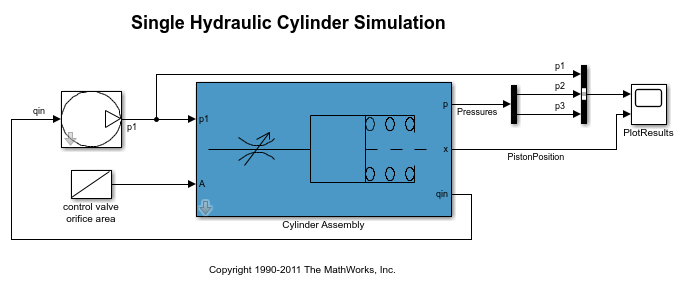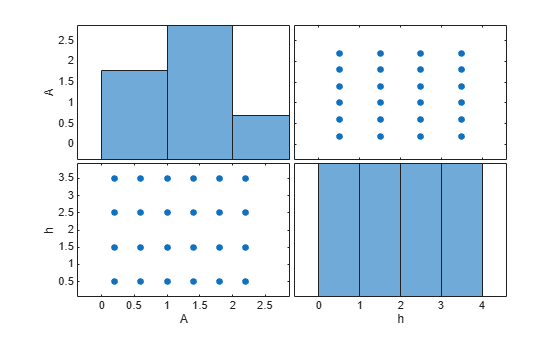# sdo.sample

Generate parameter samples for sensitivity analysis

## Syntax

``x = sdo.sample(ps)``
``x = sdo.sample(ps,N)``
``x = sdo.sample(ps,N,opt)``

## Description

example

````x = sdo.sample(ps)` generates samples using the specified parameter space definition, `ps`. If `ps` is an `sdo.ParameterSpace` object, then `sdo.sample` returns a table of samples drawn from the probability distributions specified in the `ParameterDistributions`, `RankCorrelation`, and `Options` properties of `ps`. If `ps` is an `sdo.GriddedSpace` containing only gridded parameters, then `sdo.sample` generates samples across the specified grid. If `ps` is an `sdo.GriddedSpace` containing a random-parameter subspace, then `sdo.sample` generates a mixture of gridded and random samples.```

example

````x = sdo.sample(ps,N)` specifies the number of random samples to generate.If `ps` is an `sdo.ParameterSpace` object, then `x` is a table with `N` rows and `Np` columns, where `Np` is the number of parameters in `ps`. If `ps` is an `sdo.GriddedSpace` containing only gridded parameters, then `sdo.sample` ignores `N` and returns samples across the grid as specified in the gridding options of `ps`. If `ps` is an `sdo.GriddedSpace` containing a random-parameter subspace, then `sdo.sample` generates `N` samples for each random parameter and combines them with the gridded parameters, as specified in the gridding options. ```

example

````x = sdo.sample(ps,N,opt)` specifies sampling options such as the sampling method.```

## Examples

collapse all

Open the model.

```open_system('sdoHydraulicCylinder'); ```Obtain the parameters from the model.

```p = sdo.getParameterFromModel('sdoHydraulicCylinder',{'Ac','K'}); ```

Create an `sdo.ParameterSpace` object to specify the sample distributions.

```ps = sdo.ParameterSpace(p); ```

Generate samples for the parameters.

```x = sdo.sample(ps); ```

Open the model.

```open_system('sdoHydraulicCylinder'); ```Obtain the parameters from the model.

```p = sdo.getParameterFromModel('sdoHydraulicCylinder',{'Ac','K'}); ```

Create an `sdo.ParameterSpace` object to specify the sample distributions.

```ps = sdo.ParameterSpace(p); ```

Generate 50 samples for the parameters.

```x = sdo.sample(ps,50); ```

Since R2023a

This example uses the `sdoCSTR` model discussed in detail in Design Exploration Using Parameter Sampling (Code). Among the parameters in this model are the reactor cylinder area `A` and height `h`. Suppose that you want to test the sensitivity of your design objectives over a grid of values for these parameters. To start, open the model and create `param.Continuous` objects to represent the two parameters.

```mdl = "sdoCSTR"; open_system(mdl); p = sdo.getParameterFromModel(mdl,{'A','h'});```

Specify the grid of values for each parameter by placing the values in a cell array. The grids in this example are uniformly spaced, but you can also use nonuniformly spaced values.

```Avals = {0.2 0.6 1.0 1.4 1.8 2.2}; hvals = {0.5 1.5 2.5 3.5};```

Define the gridded parameter space using this grid.

`gp = sdo.GriddedSpace(p,{Avals,hvals});`

Sample the parameter space to generate all the combinations to use for sensitivity analysis. By default, `sdo.sample` generates an exhaustive list of all possible combinations in the parameter space, `(Avals,hvals)` = (0.2,0.5), (0.6,0.5), (1.0,0.5), ..., (1.4,3.5), (1.8,3.5), (2.2,3.5). Thus, the sampled values make up a table of 24 rows.

`values = sdo.sample(gp)`
```values=24×2 table A h ___ ___ 0.2 0.5 0.6 0.5 1 0.5 1.4 0.5 1.8 0.5 2.2 0.5 0.2 1.5 0.6 1.5 1 1.5 1.4 1.5 1.8 1.5 2.2 1.5 0.2 2.5 0.6 2.5 1 2.5 1.4 2.5 ⋮ ```

You can visualize the sampled values for a view of the distribution of samples.

` sdo.scatterPlot(values)`Use `values` with `sdo.evaluate` to evaluate your design objectives at the sampled parameter values. For instance, if you have defined a cost function `design.m` to capture your design objectives, you can use `values` in a call to `sdo.evaluate` as follows.

`[yR,infoR] = sdo.evaluate(@design,p,values); `

Since R2023a

If your model contains some parameters you want to sample across a grid and others from which you want to draw random samples, you can combine gridded and random parameter spaces.

This example uses the `sdoCSTR` model discussed in detail in Design Exploration Using Parameter Sampling (Code). Open the model.

```mdl = "sdoCSTR"; open_system(mdl)```

Among the parameters in this model are the reactor cylinder area `A`, height `h`, and the feed temperature and concentration, `FeedCon0` and `FeedTemp0`. Suppose that you want to explore the sensitivity of your design constraint to these four parameters, sampling across a grid of `A` and `h` values while drawing `FeedCon0` and `FeedTemp0` from random distributions.

First, define a gridded parameter space for `A` and `h`. Use `sdo.getParameterFromModel` to create an array of `param.Continuous` objects representing `A` and `h`. Then, specify values for the grid, and create the parameter space.

```pg = sdo.getParameterFromModel(mdl,{'A','h'}); Avals = {0.2 0.6 1.0 1.4 1.8}; hvals = {0.5 1.5 2.5}; gspace = sdo.GriddedSpace(pg,{Avals,hvals});```

Next, define a random parameter space for `FeedCon0` and `FeedTemp0`. Create an array of `param.Continuous` objects and specify the probability distributions for each parameter. For this example, assign both variables a normal distribution where the mean is the current parameter value in the model and the variance is 5% of the mean. Then, create the parameter space.

```pr = sdo.getParameterFromModel(mdl,{'FeedCon0','FeedTemp0'}); distCon = makedist('normal',pr(1).Value,0.05*pr(1).Value); distTemp = makedist('normal',pr(2).Value,0.05*pr(2).Value); rspace = sdo.ParameterSpace(pr); rspace = setDistribution(rspace,'FeedCon0',distCon); rspace = setDistribution(rspace,'FeedTemp0',distTemp);```

Finally, combine the two parameter spaces into a new parameter space.

`cspace = combine(gspace,rspace)`
```cspace = GriddedSpace with properties: ParameterValues: {{1x5 cell} {1x3 cell} [1x1 prob.NormalDistribution] [1x1 prob.NormalDistribution]} Notes: [] Spaces: {[1x1 sdo.GriddedSpace] [1x1 sdo.ParameterSpace]} ParameterNames: {'A' 'h' 'FeedCon0' 'FeedTemp0'} Options: [1x1 sdo.GriddingOptions] ```

The combined space is represented by a `sdo.GriddedSpace` object. The property `cspace.Spaces` shows that `cspace` contains the two subspaces, one gridded space and one random parameter space. The property `cspace.ParameterValues` shows that the space includes the finite grid for `A` and `H`, and the probability distributions for the random variables `FeedCon0` and `FeedTemp0`.

You can now use `sdo.sample` to draw sets of sampled values from the combined space. By default, the samples are exhaustive over the grid, meaning every possible combination of grid values is included in the sampled set. Suppose that for each combination, you want to sample the random parameters ten times. Provide that value to `sdo.sample` in the `NumSample` argument.

```cspace.Options.Method = 'exhaustive'; NumSample = 10; evalues = sdo.sample(cspace,NumSample)```
```evalues=150×4 table A h FeedCon0 FeedTemp0 ___ ___ ________ _________ 0.2 0.5 10.269 275.09 0.6 0.5 10.269 275.09 1 0.5 10.269 275.09 1.4 0.5 10.269 275.09 1.8 0.5 10.269 275.09 0.2 1.5 10.269 275.09 0.6 1.5 10.269 275.09 1 1.5 10.269 275.09 1.4 1.5 10.269 275.09 1.8 1.5 10.269 275.09 0.2 2.5 10.269 275.09 0.6 2.5 10.269 275.09 1 2.5 10.269 275.09 1.4 2.5 10.269 275.09 1.8 2.5 10.269 275.09 0.2 0.5 10.917 339.77 ⋮ ```

Here, for each of the fifteen combinations of (`A`, `h`) values, `sdo.sample` assigns ten random values for (`Feedcon0`, `FeedTemp0`), resulting in 150 sets of (`A`, `h`, `FeedCon0`, `FeedTemp0`) samples.

You can also have `sdo.sample` choose only one random (`FeedCon0`,`FeedTemp0`) value for each (`A`,`h`) pair. To do so, set the sampling method of `cspace` to sequential. Note that in this case, you created `cspace` using a gridded space `gspace` that had `gspace.Options.Method = 'exhaustive'`. Thus, when you sample `cspace`, the (`A`,`h`) pairs are still exhaustive.

```cspace.Options.Method = 'sequential'; svalues = sdo.sample(cspace)```
```svalues=15×4 table A h FeedCon0 FeedTemp0 ___ ___ ________ _________ 0.2 0.5 9.5682 296.27 0.6 0.5 10.039 273 1 0.5 9.3929 284.05 1.4 0.5 9.4432 279.34 1.8 0.5 9.9966 329.67 0.2 1.5 10.766 285.92 0.6 1.5 9.6152 306.03 1 1.5 10.186 292.16 1.4 1.5 9.8872 308.11 1.8 1.5 10.559 283.72 0.2 2.5 9.4555 274.32 0.6 2.5 10.016 274.02 1 2.5 10.276 302.2 1.4 2.5 10.55 292.38 1.8 2.5 10.772 292.11 ```

Use `values` with `sdo.evalute` to evaluate your design objectives at each of the sampled parameter values. For instance, if you have defined a cost function `design`, you can use `values` in a call to `sdo.evluate` as follows.

`[yR,infoR] = sdo.evaluate(@design,p,values); `

Open the model.

```open_system('sdoHydraulicCylinder'); ```Obtain the parameters from the model.

```p = sdo.getParameterFromModel('sdoHydraulicCylinder',{'Ac','K'}); ```

Create an `sdo.ParameterSpace` object to specify the sample distributions.

```ps = sdo.ParameterSpace(p); ```

Specify the sampling method as Latin hypercube.

```opt = sdo.SampleOptions; opt.Method = 'lhs'; ```

Generate 50 samples for the parameters using Latin hypercube sampling.

```x = sdo.sample(ps,50,opt); ```

## Input Arguments

collapse all

Parameter space to sample, specified as an `sdo.ParameterSpace` object (for random sampling) or an `sdo.GriddedSpace` object (for gridded sampling or a mixture of random and gridded sampling).

Number of random samples to generate, specified as a positive integer.

Ideally, use the smallest number of samples that yield useful results, because each sample requires a model evaluation. As the number of parameters increases, the number of samples needed to explore the design space generally increases. For correlation or regression analysis, consider using `10*Np` samples, where `Np` is the number of sampled parameters.

`N` applies only to randomly sampled parameters, that is, parameters specified in an `sdo.ParameterSpace` object or an `sdo.ParameterSpace` subspace of a composite `sdo.GriddedSpace` object. `sdo.sample` ignores `N` for parameters configured for gridded sampling and instead returns samples over the specified grid values.

Example: `100`

Sampling options, specified as an `sdo.SampleOptions` or `sdo.GriddingOptions` object.

• `sdo.SampleOptions` — Specify the method for drawing samples from probability distributions defined in an `sdo.ParameterSpace` object.

• `sdo.GriddingOptions` — Specify the method for drawing samples from the grid of parameter values defined in an `sdo.GriddedSpace` object.

## Output Arguments

collapse all

Parameter samples, returned as a table.

• If `ps` is an `sdo.ParameterSpace` object, then `x` is a table with `N` rows and `Np` columns, where `Np` is the number of parameters in `ps`. Each column corresponds to a parameter and each row corresponds to a sample of the parameters. If `N` is omitted, `sdo.sample` uses `N` `= 2*Np + 1`.

• If `ps` is an `sdo.GriddedSpace` containing only gridded parameters, then `sdo.sample` ignores `N` and returns samples across the grid, as specified in the gridding options of `ps`.

• If `ps` is an `sdo.GriddedSpace` containing a random-parameter subspace, then `sdo.sample` generates `N` samples for each random parameter and combines them with the gridded parameters, as specified in the gridding options.

## Version History

Introduced in R2014a

expand all